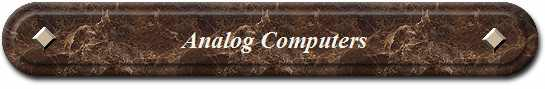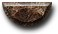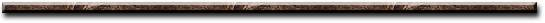News Science Technology Contact Index
 An analog computer is a mechanical, electrical, or electronic computer that performs arithmetical operations by using some continuous physical quantity, to represent numbers. They simulate continuous inputs as movements on a dial, scope, chart or graph. The mechanical analog computer uses mechanical movement to represent continuous inputs that perform mechanical operations (motion, pressure, and force). (Examples: clock, speedometer, flow meter, pressure gage, liquid level, weight scale. The electric analog computer uses mechanical movement to represent continuous inputs that perform electrical operations with sensors (voltage, current, motion, light, magnetic flux). (Examples: voltmeter, current meter, flux meter, sound meter light meter, seismograph). The electronic analog computer uses mechanical movement to represent continuous inputs that perform electronic operations with Op Amps (add, subtract, multiply, divide, integrate, differentiate or invert). (Examples: radiation detector, spectrometer)[News] [Science] [Technology] [Contact] [Index]
 Contact email: qooljaq@qooljaq.com